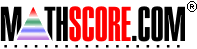Math Practice Online > free > lessons > Florida > 6th grade > Scientific Notation

## Scientific Notation

This topic covers translating between scientific and normal notation.

 Sample Problems for Scientific Notation Lesson for Scientific Notation

### This topic aligns to the following state standards

Grade 7: Num 4. Converts a number expressed in one form to its equivalent in another form.
Grade 8: Num 3. Knows equivalent forms of large and small numbers in scientific and standard notation.
Grade 7: Num 3. Expresses numbers greater than one in scientific notation.
Grade 7: Num 4. Expresses numbers in scientific notation as numbers in standard form.
Grade 8: Num 2. Expresses numbers in scientific or standard notation including decimals between 0 and 1.
Grade 9: Num 4. understands that numbers can be represented in a variety of equivalent forms, including integers, fractions, decimals, percents, scientific notation, exponents, radicals, absolute value, and logarithms

Copyright Accurate Learning Systems Corporation 2008.